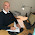## onsdag 7 oktober 2020

### 2020 Physics Nobel Prize Motivation

Roger Penrose is awarded half of the 2020 Nobel Prize in Physics

• for the discovery that black hole formation is a robust prediction of the general theory of relativity.
Let us try to analyse this (seemingly cryptic) motivation. It starts with a discovery of something, but what is that Penrose has discovered? And it ends with a robust prediction of something, but what is that is so robustly predicted? We seek answers in the theoretical foundation presented by the Academy:
• Penrose proceeded to prove that once a trapped surface had formed, it is impossible, within the theory of general relativity and with a positive energy density, to prevent the collapse towards a singularity (Penrose 1965).
• Penrose’s result is heralded as the first post-Einsteinian result in general relativity.
It appears that what Penrose has proved by logical reasoning within the mathematical general theory of relativity, is that, under some conditions,
• it is impossible to prevent collapse towards a singularity.
Questions: In what sense is this a discovery with physical meaning? Does it is impossible to prevent have a physical meaning? Does collapse towards a singularity have a physical meaning when a singularity has no meaning? Is the robustness connected to the use of logic?  Can a mathematical singularity have some internal physical structure, or is it not a singularity if so?

Remark 1: The solution of a differential equation can develop a singularity in finite time, like the function x(t) = 1/(1-t), which solves dx/dt = x*x for 0<t<1 with x(0) = 1, and x(t) tends to infinity as t approaches 1 where the solution ceases to exist. But in physics things cannot become infinite and simply cease to exist, and so one can say that the equations dx/dt = x*x cannot describe any physical reality. To discover that 1/(1-t) has a singularity for t = 1 cannot be viewed as a discovery of some physics, only a consequence of some mathematics.

Remark 2: Einstein's equations have such a cryptic formulation that solutions cannot be found in any form of generality, analytically or computationally. To predict what solutions will do under such conditions appears utterly difficult or rather impossible. If this is what Penrose actually has done, he should have the whole Prize. Or why did he not get the Prize long ago with Stephen Hawking?

PS1 Here Penrose connects to the 2nd law of thermodynamics with a black hole swallowing entropy!

PS2 Penrose claims emphatically that quantum mechanics is inconsistent, or more precisely not a theory about physics! What then about relativity!

PS3 Penrose area or science is presented to be mathematical physics. So what is this? It is not mathematics, because it lacks the rigour and strict logic of mathematics. It is not physics, because it is focussed on mathematical models per se and experimental verification is secondary. So what is it then?

• His 1965 paper actually used a proof by contradiction. He derived five properties needed in order for the system to avoid forming a singularity. Then he showed they are mutually inconsistent—a proof by contradiction.
Now proofs by contradiction have been regarded with suspicion through the history of mathematics, with constructive mathematics as mathematics without proofs by contradiction.  Many proofs by contradiction are proofs of existence of something, such as existence of a solution to some equation. Such proofs does not exhibit a solution, only says that there is one, while a constructive proof constructs/exhibits the solution, and thus contains much more concrete information.

To give an example consider this proof of the existence of God, which once was very convincing to people with big brains: God is by definition almigty. A property of almigtiness is existence, since almigtiness without existence would be a contradiction. Hence God exists. End of proof.

Do you buy it? So to be sure that black holes do exist, it would be more convincing with a constructive proof showing how they are formed.

Compare a Nobel Prize to a proof by contradiction of: there are planetary systems, with a Prize to the discovery of: how planetary systems are formed.

#### 2 kommentarer:

1.Please also see today's post in my blog Dreamheron Chronicles.
https://dreamheron.wordpress.com/2020/10/07/2020-nobel-prize-in-physics-falsifies-the-firmament/

2.Very well said, professor Claes Johnson!